### R: Image Analysis using EBImage

Currently, I am taking Statistics for Image Analysis on my masteral, and have been exploring this topic in R. One package that has the capability in this field is the EBImage from Bioconductor, which will be showcased in this post.

### Installation

For those using Ubuntu, you may likely to encounter this error:

It has something to do with the tiff.h C header file, but it's not that serious since mytechscribblings has an effective solution for this, do check that out.

### Importing Data

To import a raw image, consider the following codes:Output of display(Image).
Yes, this is the photo that we are going to use for our analysis. Needless to say, that's me and my friends. In the proceeding section we will do image manipulation and other processing.

### Image Properties

So what do we get from our raw image? To answer that, simply run print(Image). This will return the properties of the image, including the array of pixel values. With these information, we apply mathematical and statistical operations to do enhancement on the image.

There are two sections (Summary and array of the pixels) in the above output, with the following entries for the first section:

CodeValueDescription
Table 1: Information from 1st section of print(Image).
colormodeColorThe type (Color/Grayscale) of the color of the image.
storage.modedoubleType of values in the array.
dim1984 1488 3Dimension of the array, (x, y, z).
nb.total.frames:3Number of channels in each pixel, z entry in dim.
nb.render.frames1Number of channels rendered.

The second section is the obtained values from mapping pixels in the image to the real line between 0 and 1 (inclusive). Both extremes of this interval [0, 1], are black and white colors, respectively. Hence, pixels with values closer to any of these end points are expected to be darker or lighter, respectively. And because pixels are contained in a large array, then we can do all matrix manipulations available in R for processing.

It is better to start with the basic first, one of which is the brightness. As discussed above, brightness can be manipulated using + or -:

LighterDarker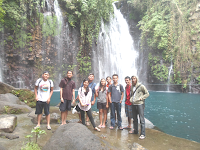Output of display(Image1).Output of display(Image2).

Contrast can be manipulated using multiplication operator(*):

LowHighOutput of display(Image3).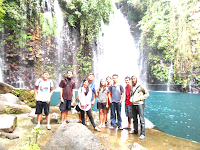Output of display(Image4).

### Gamma Correction

Gamma correction is the name of a nonlinear operation used to code and decode luminance or tristimulus values in video or still image systems, defined by the following power-law expression: \begin{equation}\nonumber V_{\mathrm{out}} = AV_{\mathrm{in}}^{\gamma} \end{equation} where $A$ is a constant and the input and output values are non-negative real values; in the common case of $A = 1$, inputs and outputs are typically in the range 0-1. A gamma value $\gamma< 1$ is sometimes called an encoding gamma (Wikipedia, Ref. 1).

$\gamma = 2$$\gamma = 0.7$Output of display(Image5).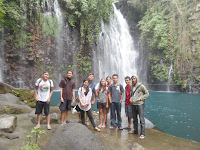Output of display(Image6).

### Cropping

Slicing array of pixels, simply mean cropping the image.Output of the above code.

### Spatial Transformation

Spatial manipulation like rotate (rotate), flip (flip), and translate (translate) are also available in the package. Check this out,### Color Management

Since the array of pixels has three axes in its dimension, for example in our case is 1984 x 1488 x 3. The third axis is the slot for the three channels: Red, Green and Blue, or RGB. Hence, transforming the color.mode from Color to Grayscale, implies disjoining the three channels from single rendered frame (three channels for each pixel) to three separate array of pixels for red, green, and blue frames.

OriginalRed Channel
Table 5: Color Mode Transformation.Green ChannelBlue Channel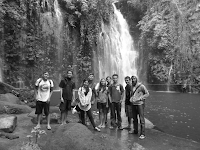To revert the color mode, simply run

### Filtering

In this section, we will do smoothing/blurring using low-pass filter, and edge-detection using high-pass filter. In addition, we will also investigate median filter to remove noise.

Low-Pass (Blur)
Table 6: Image Filtering.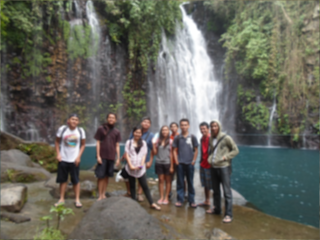High PassOriginalFiltered
Table 7: Median Filter.From Google, Link Here.Output of display(medFltr)

For comparison, I run median filter on first-neighborhood in Mathematica, and I got thisClearly, Mathematica has better enhancement than R for this particular filter. But R has a good foundation already, as we witness with EBImage. There are still lots of interesting functions in the said package, that is worth exploring, I suggest you check that out.

For the meantime, we will stop here, but hoping we can play more on this topic in the succeeding post.

### References

1. Gamma Correction. Wikipedia. Retrieved August 31, 2014.
2. Gregoire Pau, Oleg Sklyar, Wolfgang Huber (2014). Introduction to EBImage, an image processing and analysis toolkit for R.